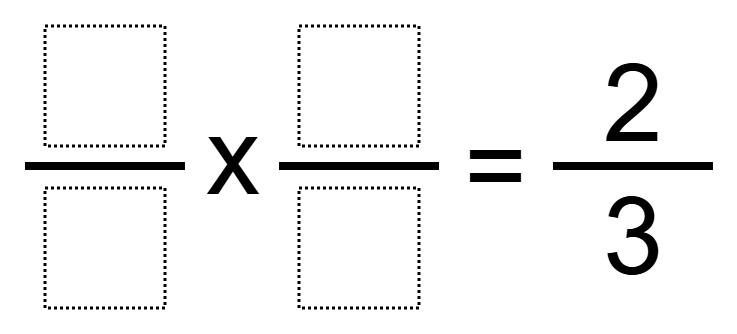# Multiplying Fractions 5

Directions: Using the digits 1 to 9 at most one time each, place a digit in each box to make a true equation.### Hint

How can there be more than one way to make a product of 2/3?

There are many possible answers including 4/3 x 1/2.

Source: Robert Kaplinsky

## Add Fractions with Decimal Sums

Directions: Using the digits 1 to 9 at most one time each, place a digit …

1.•The integer of 2 is written as 2/1 as a fraction. This means that you will be using the value of 1 twice.

•you used the 1 twice rethink it

2.•The integer of 2 is written as 2/1 as a fraction. This means that you will be using the value of 1 twice.

3.4.5.2/6 x 8/4= 16/24 or 2/3